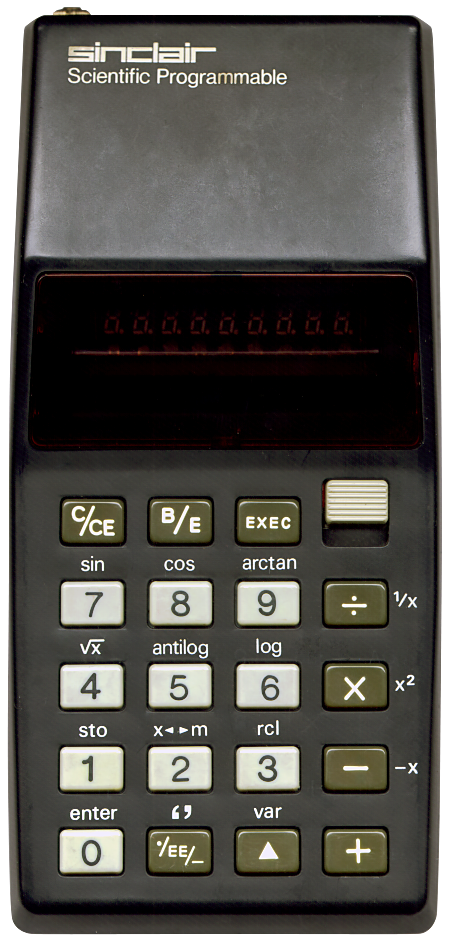# sinclair Scientific Programmable

## Description

 Brand: sinclair Model: Scientific Programmable Type: Programmable scientific calculator Picture:Batteries: 9V x 1 Lifetime: Introduced: 1975 Terminated: unknown Notes: A calculator designed by Clive Sinclair's famous English brand for cars, computers and calculators. Made in England. This calculator is very different from any other scientific programmable RPN calculator I have ever seen. It is simply impossible to use it without first reading its 16-page manual. It took me a good five minutes to figure out how to even multiply two numbers when I first held it in my hands. It is a calculator with some very peculiar features: The calculator has a 9 segment display. The first segment is used for the negative sign, the last three for the exponent and its sign. Values are always displayed using scientific notation. So, the number "42" will be displayed as "4.2000   1". After the first value (as common with RPN calculators) the 'enter' key must be pressed. Since the 'enter'-key is above the '0' key, the '▲'-key (shift) needs to be pressed first. So, when multiplying 6 by 9 one needs to press: '6', '▲', '0' (='enter'), '9', 'x'. According to the manual using 'enter' is mandatory. I have however found out that the '+' key can be used as well, so to calculate 6 x 9 one can also press: '6', '+', '9', 'x'. This works because the first '+' adds 6 to the 0 on the stack. Only works when the 'C/CE'-key is pressed first. There is just one stack position. Some keys have multiple functions. For instance, after pressing '6', '▲', '0' (='enter'), pressing the 'x'-key will display the square of 6. Entering negative numbers needs some training. For instance, the number "-5", at the start of a calculation (after first pressing the 'C/CE'-key) can be entered by pressing '5' followed by '-'. This also probably works because this substracts 5 from the 0 on the stack. When in the middle of a calculation one needs to press '▲' and '-' to negate a number. One key is really special, the './EE/-'-key. What it does depends on how many times you press it. Press once to type decimals. Press twice to enter the exponent, press three times to change the sign of the exponent to negative. Division by zero gives the result "0.0000". Square roots of negative numbers display the root of their non-negative value. Same for logarithms. Considering trigonometric functions. The calculator has Radians-based "sin", "cos" and "artan". The manual explains how to calculate "arcsin", "arccos" and "tan" using formulae. Since the calculator is programmable the manual also mentions that "A program can be written to provide any of these functions as a single keystroke operation." The accepted input values for "sin" and "cos" must be between 0 and π/2. Other values might produce (unpredictable?) results or will hang the calculator. Now, programming. The calculator has 24 programming steps. All programs must start and end by pressing the 'B/E'-key (Begin/End). When entering a program, the number keys perform their shifted functions. Use the 'var'-key to display a value. To enter a number (integer! no decimals allowed) one presses the '‘’'-key before and after the number. So, to enter a simple program to multiply any number by five, enter: 'C/CE', 'B/E', '0' (='enter'), '‘’', 5, '‘’', 'x', '▲' (='var'), and 'B/E'. Press the 'C/CE'-key to leave programming mode. Press any number followed by the 'exec'-key to now multiply it by 5. All in all it is quite a feat to produce a small pocket size programmable calculator. I still remember seeing Tandy's version, the Radio Shack EC‑4001 in a Tandy catalogue and being utterly amazed by it. So capable with so few buttons! Takes some getting used to however. Many thanks to Richard Pilkington of the University of Salford for donating this calculator to the museum.